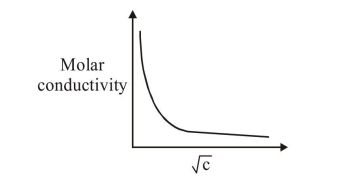# The variation of molar conductivity with concentration of an electrolyte (X) in aqueous solution is shown in the given figure.

Question:

The variation of molar conductivity with concentration of an electrolyte (X) in aqueous solution is shown in the given figure.The electrolyte $\mathrm{X}$ is :

1. $\mathrm{CH}_{3} \mathrm{COOH}$

2. $\mathrm{KNO}_{3}$

3. $\mathrm{HCl}$

4. $\mathrm{NaCl}$

Correct Option: 1

Solution:

Its a weak electrolyte hence : $\mathrm{CH}_{3} \mathrm{COOH}$